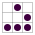# D码点评:17 补码之美``````X - Y = X + (-Y)
``````

## 原码

1 和 0. 于是,在计算机里,我们采用二进制来表示整数.

``````1 - 1 = 1 + (-1) = 0001 + 1001 = 1010 = -2
``````

## 补码

``````-1  = 0000 - 0001

= 10000 - 0001  (高位借一)

= (1111 + 0001) - 0001

= (1111 - 0001) + 0001(第一步:取反)

= 1110 + 0001(第二步:加一)

= 1111
``````

``````-Y = 0000 - Y

= (1111 + 1 ) - Y

= (1111 - Y) + 1   将正整数 Y 的二进制先取反,再加一
``````

## 简单之美

``````1. 数轴上,左边的数永远小于右边的数. 比如 -8 < -2 < 0 < 3 < 5
2. 数轴上,只有一个零.
3. 相邻整数之间相差一.
``````

``````1000    -8
1001    -7
1010    -6
1011    -5
1100    -4
1101    -3
1110    -2
1111    -1
0000     0
0001     1
0010     2
0011     3
0100     4
0101     5
0110     6
0111     7
1000     8
``````
1. 只看负数的补码表示,明显 1000 < 1001,补码表示下,左边的负数很自然小于右边的负数.

2. 很明显只有一个零,就是 0000,不存在 -0 跟 0 的表示不一样.

3. 再看相邻整数之间,左边的整数加一,就是右边的整数,包括 1111 + 1 = 0000 .

(完)

## 巡阅

2014-02-11

### 加入 珠海GDG

1. 注册 Meetup
2. 关注 Zhuhai GDG
3. 或扫描:```    第一时间获知谷歌最新技术,
可以学到如何去谷歌平台上赚钱的思路和方法,
可以认识很多有可能将来一起走上自己创业道路的人,
可以学会把你的创新带向国际市场,
参加那里的活动有经常和国际上的开发者们进行交流的机会...
```

#### PPS:

```0. 有趣 ~ 至少是自个儿有兴趣的领域吧...
1. 有料 ~ 至少有点儿原创的东西吧..
2. 有种 ~ 至少不能是成功学吧!
```

(发空邮件到 [email protected] 即完成订阅)

### 微信公号

```    G术图书 (gb:推荐好书,书无中外)
D码点评 (dd:麻辣评点,善意满盈)
G说公论 (gt:时评杂文,新旧不拘)
珠的自白(dm:大妈自述,每周一篇)
海选文章(hd:得要相信,大妈法眼)
```

#### 订阅方法

• 搜索微信号 `GDG-ZhuHai`
• 或查找公众号: `GDG珠海`
• 或扫描:### GDG珠海 社区资源:

Zoom.Quiet’s Chaos42 | Substack### 蟒营®编程思维提高班Python版

**2021.01.11** 因大妈再次创业暂停定期开设, 转换为预约触发:
• + 任何问题, 随时邮件提问可也:
`[email protected]`